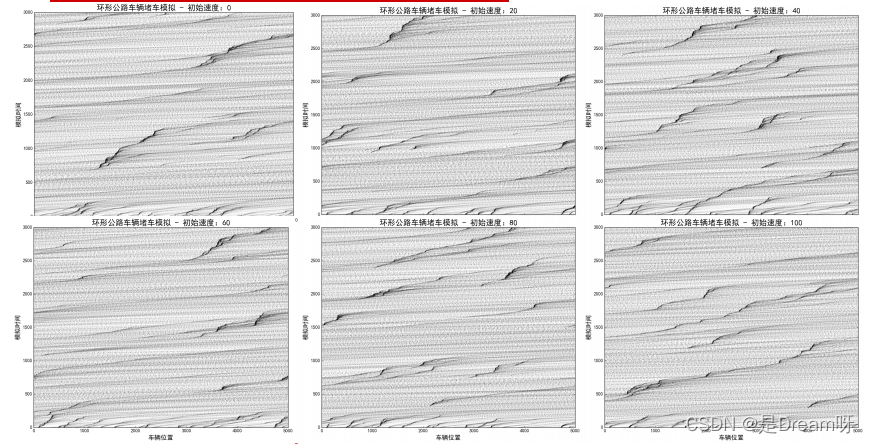# 公路堵车概率模型：Nagel-Schreckenberg 模型模拟

📢📢📢📣📣📣 🌻🌻🌻Hello，大家好我叫是Dream呀，一个有趣的Python博主，多多关照😜😜😜 🏅🏅🏅Python领域优质创作者，欢迎大家找我合作学习（文末有VX 想进学习交流群or学习资料 欢迎+++） 💕` 入门须知：这片乐园从不缺乏天才，努力才是你的最终入场券！🚀🚀🚀` 💓`最后，愿我们都能在看不到的地方闪闪发光，一起加油进步🍺🍺🍺` 🍉🍉🍉“一万次悲伤，依然会有Dream，我一直在最温暖的地方等你”，唱的就是我！哈哈哈~🌈🌈🌈 🌟🌟🌟✨✨✨# Nagel-Schreckenberg交通流模型

```import numpy as np
import matplotlib as mpl
import matplotlib.pyplot as plt
def clip(x, path):
for i in range(len(x)):
if x[i] >= path:
x[i] %= path
if __name__ == "__main__":
mpl.rcParams['font.sans-serif'] = ['Fangsong']  # 设置字体
mpl.rcParams['axes.unicode_minus'] = False  # 不显示负号坐标
path = 5000     # 环形公路的长度
n = 100         # 公路中的车辆数目
v0 = 50          # 车辆的初始速度
p = 0.3         # 随机减速概率
Times = 3000
np.random.seed(0)
x = np.random.rand(n) * path
x.sort()
v = np.tile([v0], n).astype(np.float)
plt.figure(figsize=(9, 7), facecolor='w')
for t in range(Times):
plt.scatter(x, [t]*n, s=1, c='k', alpha=0.05)
for i in range(n):
if x[(i+1)%n] > x[i]:
d = x[(i+1) % n] - x[i]   # 距离前车的距离
else:
d = path - x[i] + x[(i+1) % n]
if v[i] < d:
if np.random.rand() > p:
v[i] += 1
else:
v[i] -= 1
else:
v[i] = d - 1
v = v.clip(0, 150)
x += v
clip(x, path)
plt.xlim(0, path)
plt.ylim(0, Times)
plt.xlabel('车辆位置', fontsize=14)
plt.ylabel('模拟时间', fontsize=14)
plt.title('环形公路车辆堵车模拟', fontsize=18)
plt.show()```

# 初始车速对NS模型的影响# 减速概率对NS模型的影响

❤️❤️❤️如果你喜欢的话，就不要吝惜你的一键三连了~

### 最后编辑：2022/8/3编辑：xuanyun